# Constraints and joints¶

A constraint describes how two bodies interact with each other. Constraints can be simple joints, which allow bodies to pivot around each other, as well as springs, grooves or motors.

## Pin joint¶

The pin joint links two bodies with a solid bar or pin.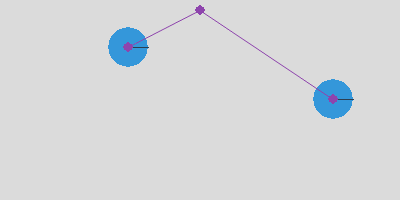We create a new `PinJoint` class which connects the two bodies `b` and `b2` at their anchor points `a` and `a2` via a pin joint. By adding the new joint directly to `space`, we save one line of code.

```class PinJoint:
def __init__(self, b, b2, a=(0, 0), a2=(0, 0)):
joint = pymunk.constraint.PinJoint(b, b2, a, a2)
```

We define the static body `b0` which will be used for everything static:

```b0 = space.static_body
```

We will label position points with `p` and vectors with `v`. The suspension point for the pendulum is `p` and the initial pin vector is `v`:

```p = Vec2d(200, 190)
v = Vec2d(80, 0)
```

Now we can define the first circular body `c` and attach it with a pin joint to the static body `b0` at position `p`:

```c = Circle(p+v)
PinJoint(b0, c.body, p)
```

A second circular body `c2` is placed at twice the vector distance:

```c2 = Circle(p+2*v)
PinJoint(b0, c2.body, p)
```

The two pendulums swing at different frequencies.

`pin1.py`

```# two pendulums of different length
from joint import *

p = Vec2d(200, 190)
v = Vec2d(80, 0)

c = Circle(p+v)
PinJoint(b0, c.body, p)

c2 = Circle(p+2*v)
PinJoint(b0, c2.body, p)

App().run()
```

## Double pendulum¶

The double pendulum is a pendulum linked to another one. Together they execute a complicated chaotic movement.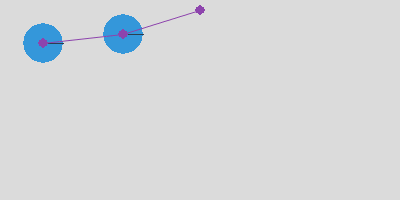The first segment is identical to the previous one:

```c = Circle(p+v)
PinJoint(b0, c.body, p)
```

The second segment is attached to the first circular disc:

```c2 = Circle(p+2*v)
PinJoint(c.body, c2.body)
```

The two pendulums create a complicated movement.

`pin2.py`

```# double pendulum
from joint import *

p = Vec2d(200, 190 )
v = Vec2d(80, 0)

c = Circle(p+v)
PinJoint(b0, c.body, p)

c2 = Circle(p+2*v)
PinJoint(c.body, c2.body)

App().run()
```

## Pivot joint¶

A pivot joint allows two objects to pivot about a single point.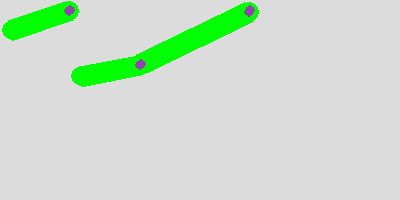We define a new `PivotJoint` class which connects the two bodies `b` and `b2` at their anchor points `a` and `a2` via a pivot joint. By adding the new joint directly to `space`, we save one line of code.

```class PivotJoint:
def __init__(self, b, b2, a=(0, 0), a2=(0, 0), collide=True):
joint = pymunk.constraint.PinJoint(b, b2, a, a2)
joint.collide_bodies = collide
```

We define the first segment with its position point `p` and its direction vector `v`. Then we define a pivot joint in the static body `b0` located at position `p`:

```segment = Segment(p, v)
PivotJoint(b0, segment.body, p)
```

A bit to the right, we create another segment, twice the length of the first:

```segment = Segment(p+3*v, 2*v)
PivotJoint(b0, segment.body, p+3*v)
```

To this longer segment we attach a shorter one to create a double pendulum:

```segment2 = Segment(p+5*v, v)
PivotJoint(segment.body, segment2.body, 2*v)
```

`joint1.py`

```# pivot point
from joint import *

p = Vec2d(70, 190)
v = Vec2d(60, 0)

segment = Segment(p, v)
PivotJoint(b0, segment.body, p)

segment = Segment(p+3*v, 2*v)
PivotJoint(b0, segment.body, p+3*v)

segment2 = Segment(p+5*v, v)
PivotJoint(segment.body, segment2.body, 2*v)

App().run()
```

## Rag doll¶

In a rag doll, the different elements of the body (torso, arm, forarm, leg) can cross, without creating collisions. This is possible when the shapes belong to the same group:

```shape.filter = pymunk.ShapeFilter(group=1)
```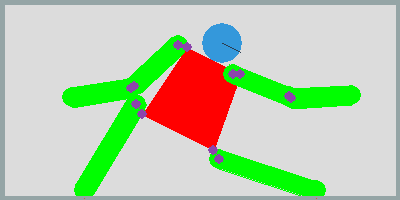We define the torse by it’s center point `p0` and the 4 vertices:

```p0 = Vec2d(200, 150)
vs = [(-30, 50), (30, 50), (40, -50), (-40, -50)]
v0, v1, v2, v3 = vs
torso = Poly(p0, vs)
c = pymunk.Circle(torso.body, 20, (0, 70))
```

Then we attach the left arm to the torso:

```arm = Segment(p0+v0, -v)
PivotJoint(torso.body, arm.body, v0, (0, 0))
```

and then the left forearm to the upper arm:

```forearm = Segment(p0+v0-v, -v)
PivotJoint(arm.body, forearm.body, -v, (0, 0))
```

We do the same on the right side, and finally attach the two legs:

```leg = Segment(p0+v2, (20, -100))
PivotJoint(torso.body, leg.body, v2, (0, 0))
```

`joint2.py`

```# rag doll
from joint import *

Box()

p = Vec2d(200, 120)
vs = [(-30, 40), (30, 40), (40, -40), (-40, -40)]
v0, v1, v2, v3 = vs
torso = Poly(p, vs)

c = pymunk.Circle(torso.body, 20, (0, 60))

v = Vec2d(60, 0)
arm = Segment(p+v0, -v)
PivotJoint(torso.body, arm.body, v0, (0, 0))

forearm = Segment(p+v0-v, -v)
PivotJoint(arm.body, forearm.body, -v, (0, 0))

arm = Segment(p+v1, v)
PivotJoint(torso.body, arm.body, v1, (0, 0))

forearm = Segment(p+v1+v, v)
PivotJoint(arm.body, forearm.body, v, (0, 0))

leg = Segment(p+v2, (20, -100))
PivotJoint(torso.body, leg.body, v2, (0, 0))

leg = Segment(p+v3, (-10, -100))
PivotJoint(torso.body, leg.body, v3, (0, 0))

App().run()
```

## Motors¶

The `SimpleMotor` class keeps the relative angular velocity between two bodies at a constant rate.

```class SimpleMotor:
def __init__(self, b, b2, rate):
joint = pymunk.constraint.SimpleMotor(b, b2, rate)
```

In the following example code we have 3 constraints:

• a pivot joint makes a segment rotation around a point
• a pivot + motor joint, makes a rotation around a pivot point at a constant angular speed (10 radians/s)
• a motor joint, makes a freely moving segment follow the motor angle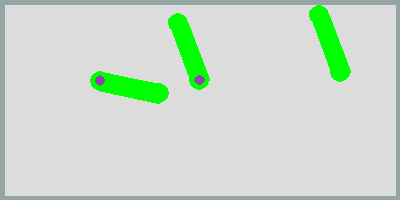This is the passive pivot joint:

```p = 100, 120
v = 80,10
arm = Segment(p, v)
PivotJoint(b0, arm.body, p)
```

This is the motorized pivot joint:

```p1 = 200, 120
arm = Segment(p1, v)
PivotJoint(b0, arm.body, p1)
SimpleMotor(b0, arm.body, 10)
```

This is the motor joint without a pivot:

```p2 = 300, 120
arm = Segment(p2, v)
SimpleMotor(b0, arm.body, 10)
```

`joint3.py`

```# motors
from joint import *
Box()

p = 100, 120
v = 60,10
arm = Segment(p, v)
PivotJoint(b0, arm.body, p)

p1 = 200, 120
arm = Segment(p1, v)
PivotJoint(b0, arm.body, p1)
SimpleMotor(b0, arm.body, 10)

p2 = 300, 120
arm = Segment(p2, v)
SimpleMotor(b0, arm.body, 10)

App().run()
```

## Motors moving at different speeds¶

In the following example 3 segments move at 3 different rotation rates. The first motor moves at speed 1:

```arm = Segment(p, v)
PivotJoint(b0, arm.body, p)
SimpleMotor(b0, arm.body, 1)
```

The second motor moves at speed 3 and the last one at speed 6, which means about one rotation per second.`joint4.py`

```# different rotation speeds
from joint import *

p = 100, 100
v = 80, 0
arm = Segment(p, v)
PivotJoint(b0, arm.body, p)
SimpleMotor(b0, arm.body, 1)

p = 200, 100
arm = Segment(p, v)
PivotJoint(b0, arm.body, p)
SimpleMotor(b0, arm.body, 5)

p = 300, 100
arm = Segment(p, v)
PivotJoint(b0, arm.body, p)
SimpleMotor(b0, arm.body, 10)

App().run()
```

## Wheeled car¶

To create a simplistic car we attach 2 wheels to a rectangular chassis, based on a central position point and a vertex list:

```p = Vec2d(200, 150)
vs = [(-50, -30), (50, -30), (50, 30), (-50, 30)]
v0, v1, v2, v3 = vs
chassis = Poly(p, vs)
```

We place the wheels to the lower left and right corners of the chassis:

```wheel1 = Circle(p+v0)
wheel2 = Circle(p+v1)
```

Both wheels are then motorized at the same speed:

```PivotJoint(chassis.body, wheel1.body, v0, (0, 0))
SimpleMotor(chassis.body, wheel1.body, 5)
```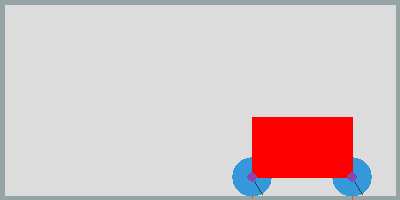`joint5.py`

```# car with pivot and motor joint
from joint import *

Box()

p = Vec2d(200, 150)
vs = [(-50, -30), (50, -30), (50, 30), (-50, 30)]
v0, v1, v2, v3 = vs
chassis = Poly(p, vs)

wheel1 = Circle(p+v0)
wheel2 = Circle(p+v1)

PivotJoint(chassis.body, wheel1.body, v0, (0, 0), False)
SimpleMotor(chassis.body, wheel1.body, 5)

PivotJoint(chassis.body, wheel2.body, v1, (0, 0), False)
SimpleMotor(chassis.body, wheel2.body, 5)

App().run()
```

## Slide joint¶

A slide joint is like a pin joint, but instead of having a fixed distance, the distance between the two anchor points can vary between a minimum and maximum distance. First we define a rotating arm created from a `Segment`. The segment is placed at position `p0` and has a direction vector `v`:

```p = Vec2d(200, 120)
v = Vec2d(80, 0)
arm = Segment(p, v)
```

In order to rotate the arm, we add to joints: a pivot joint and a simple motor joint:

```PivotJoint(b0, arm.body, p)
SimpleMotor(b0, arm.body, 1)
```

The we create a ball from the `Circle` class and attach with a `SlideJoint` to the rotating arm:

```ball = Circle(p+v+(40, 0), r)
SlideJoint(arm.body, ball.body, v, (-r, 0), min, max)
```

In this case the arm and the ball do collide. Now we create a second arm-and-ball mechanism, and this time don’t allow bodies to collide:

```ball = Circle(p+v+(40, 0), r)
SlideJoint(arm.body, ball.body, v, (-r, 0), min, max, False)
```

This is the simulation result. The first ball collides with the arm. The second ball does not collide with the moving arm.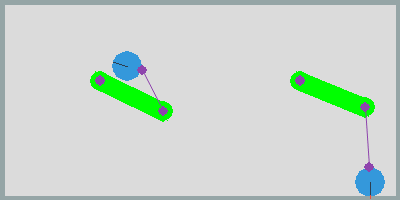`joint6.py`

## Groove joint¶

GrooveJoint is similar to a PivotJoint, but with a linear slide. First we create a rotating arm:

```arm = Segment(p, v)
PivotJoint(b0, arm.body, p)
SimpleMotor(b0, arm.body, 1)
```

Then we create a circle and attach it to a groove joint:

```ball = Circle(p+v, 20)
GrooveJoint(arm.body, ball.body, (0, 0), v, (0, 0))
```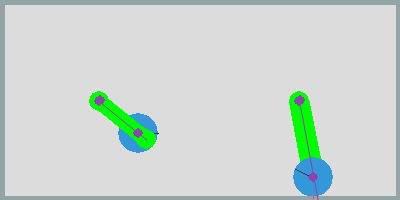`joint7.py`

## Damped rotary spring and rotary limit joint¶

To simplify its use we define again two new classes.

```class DampedRotarySpring:
def __init__(self, b, b2, angle, stiffness, damping):
joint = pymunk.constraint.DampedRotarySpring(
b, b2, angle, stiffness, damping)
```

and

```class RotaryLimitJoint:
def __init__(self, b, b2, min, max, collide=True):
joint = pymunk.constraint.RotaryLimitJoint(b, b2, min, max)
joint.collide_bodies = collide
```

Then we define a rotary segment:

```arm = Segment(p0, v)
PivotJoint(b0, arm.body, p0)
SimpleMotor(b0, arm.body, 1)
```

We attache a second arm segment via a damped rotary spring:

```arm2 = Segment(p0+v, v)
PivotJoint(arm.body, arm2.body, v, (0, 0))
DampedRotarySpring(arm.body, arm2.body, 0, 10000000, 10000)
```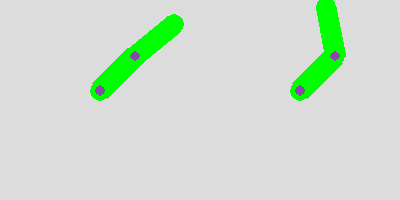`joint8.py`

## Gear joint¶

A gear joint keeps the angular velocity ratio of a pair of bodies constant.

We define two wheels who touch:

```p0 = Vec2d(200, 120)
r1, r2 = 40, 80
v = Vec2d(r1+r2, 0)
wheel1 = Circle(p0, r1)
wheel2 = Circle(p0+v, r2)
```

Then we motorize the first wheel, place a pivot on both bodies, and add a gear joint with a ratio of -r2/r1:

```SimpleMotor(b0, wheel1.body, 5)
PivotJoint(b0, wheel1.body, p0)
PivotJoint(b0, wheel2.body, p0+v)
GearJoint(wheel1.body, wheel2.body, 0, -r2/r1)
```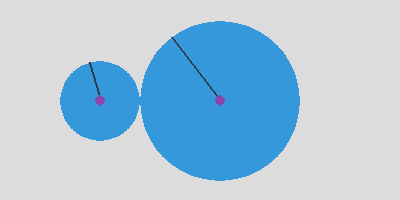`joint10.py`

## Creating GIF images¶

We can use the PIL library to create an animated GIF.

```    def make_gif(self):
if self.gif > 0:
strFormat = 'RGBA'
raw_str = pygame.image.tostring(self.screen, strFormat, False)
image = Image.frombytes(
strFormat, self.screen.get_size(), raw_str)
self.images.append(image)
self.gif -= 1
if self.gif == 0:
self.images.save('joint.gif',
save_all=True, append_images=self.images[1:],
optimize=True, duration=1000//fps, loop=0)
self.images = []
```

## Complete source code¶

`joint.py`

```import pymunk
from pymunk.pygame_util import *
from pymunk.vec2d import Vec2d

import pygame
from pygame.locals import *

import math
from PIL import Image

space = pymunk.Space()
space.gravity = 0, -900
b0 = space.static_body

size = w, h = 400, 200
fps = 30
steps = 10

BLACK = (0, 0, 0)
GRAY = (220, 220, 220)
WHITE = (255, 255, 255)

class PinJoint:
def __init__(self, b, b2, a=(0, 0), a2=(0, 0)):
joint = pymunk.constraint.PinJoint(b, b2, a, a2)

class PivotJoint:
def __init__(self, b, b2, a=(0, 0), a2=(0, 0), collide=True):
joint = pymunk.constraint.PinJoint(b, b2, a, a2)
joint.collide_bodies = collide

class SlideJoint:
def __init__(self, b, b2, a=(0, 0), a2=(0, 0), min=50, max=100, collide=True):
joint = pymunk.constraint.SlideJoint(b, b2, a, a2, min, max)
joint.collide_bodies = collide

class GrooveJoint:
def __init__(self, a, b, groove_a, groove_b, anchor_b):
joint = pymunk.constraint.GrooveJoint(
a, b, groove_a, groove_b, anchor_b)
joint.collide_bodies = False

class DampedRotarySpring:
def __init__(self, b, b2, angle, stiffness, damping):
joint = pymunk.constraint.DampedRotarySpring(
b, b2, angle, stiffness, damping)

class RotaryLimitJoint:
def __init__(self, b, b2, min, max, collide=True):
joint = pymunk.constraint.RotaryLimitJoint(b, b2, min, max)
joint.collide_bodies = collide

class RatchetJoint:
def __init__(self, b, b2, phase, ratchet):
joint = pymunk.constraint.GearJoint(b, b2, phase, ratchet)

class SimpleMotor:
def __init__(self, b, b2, rate):
joint = pymunk.constraint.SimpleMotor(b, b2, rate)

class GearJoint:
def __init__(self, b, b2, phase, ratio):
joint = pymunk.constraint.GearJoint(b, b2, phase, ratio)

class Segment:
self.body = pymunk.Body()
self.body.position = p0
shape = pymunk.Segment(self.body, (0, 0), v, radius)
shape.density = 0.1
shape.elasticity = 0.5
shape.filter = pymunk.ShapeFilter(group=1)
shape.color = (0, 255, 0, 0)

class Circle:
self.body = pymunk.Body()
self.body.position = pos
shape.density = 0.01
shape.friction = 0.5
shape.elasticity = 1

class Box:
def __init__(self, p0=(0, 0), p1=(w, h), d=4):
x0, y0 = p0
x1, y1 = p1
pts = [(x0, y0), (x1, y0), (x1, y1), (x0, y1)]
for i in range(4):
segment = pymunk.Segment(
space.static_body, pts[i], pts[(i+1) % 4], d)
segment.elasticity = 1
segment.friction = 0.5

class Poly:
def __init__(self, pos, vertices):
self.body = pymunk.Body(1, 100)
self.body.position = pos

shape = pymunk.Poly(self.body, vertices)
shape.filter = pymunk.ShapeFilter(group=1)
shape.density = 0.01
shape.elasticity = 0.5
shape.color = (255, 0, 0, 0)

class Rectangle:
def __init__(self, pos, size=(80, 50)):
self.body = pymunk.Body()
self.body.position = pos

shape = pymunk.Poly.create_box(self.body, size)
shape.density = 0.1
shape.elasticity = 1
shape.friction = 1

class App:
def __init__(self):
pygame.init()
self.clock = pygame.time.Clock()
self.screen = pygame.display.set_mode(size)
self.draw_options = DrawOptions(self.screen)
self.running = True
self.gif = 0
self.images = []

def run(self):
while self.running:
for event in pygame.event.get():
self.do_event(event)

self.draw()
self.clock.tick(fps)

for i in range(steps):
space.step(1/fps/steps)

pygame.quit()

def do_event(self, event):
if event.type == QUIT:
self.running = False

if event.type == KEYDOWN:
if event.key in (K_q, K_ESCAPE):
self.running = False

elif event.key == K_p:
pygame.image.save(self.screen, 'joint.png')

elif event.key == K_g:
self.gif = 60

def draw(self):
self.screen.fill(GRAY)
space.debug_draw(self.draw_options)
pygame.display.update()

text = f'fpg: {self.clock.get_fps():.1f}'
pygame.display.set_caption(text)
self.make_gif()

def make_gif(self):
if self.gif > 0:
strFormat = 'RGBA'
raw_str = pygame.image.tostring(self.screen, strFormat, False)
image = Image.frombytes(
strFormat, self.screen.get_size(), raw_str)
self.images.append(image)
self.gif -= 1
if self.gif == 0:
self.images.save('joint.gif',
save_all=True, append_images=self.images[1:],
optimize=True, duration=1000//fps, loop=0)
self.images = []

if __name__ == '__main__':
Box()
p = 100, 180
c = Circle(p)
c.body.apply_impulse_at_local_point((10000, 0))
App().run()
```